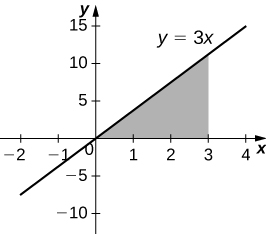$$\newcommand{\id}{\mathrm{id}}$$ $$\newcommand{\Span}{\mathrm{span}}$$ $$\newcommand{\kernel}{\mathrm{null}\,}$$ $$\newcommand{\range}{\mathrm{range}\,}$$ $$\newcommand{\RealPart}{\mathrm{Re}}$$ $$\newcommand{\ImaginaryPart}{\mathrm{Im}}$$ $$\newcommand{\Argument}{\mathrm{Arg}}$$ $$\newcommand{\norm}{\| #1 \|}$$ $$\newcommand{\inner}{\langle #1, #2 \rangle}$$ $$\newcommand{\Span}{\mathrm{span}}$$

# 6.3E: Exercises for the Shell Method

$$\newcommand{\vecs}{\overset { \rightharpoonup} {\mathbf{#1}} }$$ $$\newcommand{\vecd}{\overset{-\!-\!\rightharpoonup}{\vphantom{a}\smash {#1}}}$$$$\newcommand{\id}{\mathrm{id}}$$ $$\newcommand{\Span}{\mathrm{span}}$$ $$\newcommand{\kernel}{\mathrm{null}\,}$$ $$\newcommand{\range}{\mathrm{range}\,}$$ $$\newcommand{\RealPart}{\mathrm{Re}}$$ $$\newcommand{\ImaginaryPart}{\mathrm{Im}}$$ $$\newcommand{\Argument}{\mathrm{Arg}}$$ $$\newcommand{\norm}{\| #1 \|}$$ $$\newcommand{\inner}{\langle #1, #2 \rangle}$$ $$\newcommand{\Span}{\mathrm{span}}$$ $$\newcommand{\id}{\mathrm{id}}$$ $$\newcommand{\Span}{\mathrm{span}}$$ $$\newcommand{\kernel}{\mathrm{null}\,}$$ $$\newcommand{\range}{\mathrm{range}\,}$$ $$\newcommand{\RealPart}{\mathrm{Re}}$$ $$\newcommand{\ImaginaryPart}{\mathrm{Im}}$$ $$\newcommand{\Argument}{\mathrm{Arg}}$$ $$\newcommand{\norm}{\| #1 \|}$$ $$\newcommand{\inner}{\langle #1, #2 \rangle}$$ $$\newcommand{\Span}{\mathrm{span}}$$

For exercises 1 - 6, find the volume generated when the region between the two curves is rotated around the given axis. Use both the shell method and the washer method. Use technology to graph the functions and draw a typical slice by hand.

1) [T] Over the curve of $$y=3x,$$ $$x=0,$$ and $$y=3$$ rotated around the $$y$$-axis.

2) [T] Under the curve of $$y=3x,$$ $$x=0$$, and $$x=3$$ rotated around the $$y$$-axis.$$V = 54π$$ units3

3) [T] Over the curve of $$y=3x,$$ $$x=0$$, and $$y=3$$ rotated around the $$x$$-axis.

4) [T] Under the curve of $$y=3x,$$ $$x=0,$$ and $$x=3$$ rotated around the $$x$$-axis.$$V = 81π$$ units3

5) [T] Under the curve of $$y=2x^3,x=0,$$ and $$x=2$$ rotated around the $$y$$-axis.

6) [T] Under the curve of $$y=2x^3,x=0,$$ and $$x=2$$ rotated around the $$x$$-axis.$$V = \frac{512π}{7}$$ units3

For exercises 7 - 16, use shells to find the volumes of the given solids. Note that the rotated regions lie between the curve and the $$x$$-axis and are rotated around the $$y$$-axis.

7) $$y=1−x^2,$$ $$x=0,$$ and $$x=1$$

8) $$y=5x^3,$$ $$x=0$$, and $$x=1$$

$$V = 2π$$ units3

9) $$y=\dfrac{1}{x},$$ $$x=1,$$ and $$x=100$$

10) $$y=\sqrt{1−x^2},$$ $$x=0$$, and $$x=1$$

$$V= \frac{2π}{3}$$ units3

11) $$y=\dfrac{1}{1+x^2},$$ $$x=0$$,and $$x=3$$

12) $$y=\sin x^2,x=0$$, and $$x=\sqrt{π}$$

$$V= 2π$$ units3

13) $$y=\dfrac{1}{\sqrt{1−x^2}},$$ $$x=0$$, and $$x=\frac{1}{2}$$

14) $$y=\sqrt{x},$$ $$x=0$$, and $$x=1$$

$$V = \frac{4π}{5}$$ units3

15) $$y=(1+x^2)^3,$$ $$x=0$$, and $$x=1$$

16) $$y=5x^3−2x^4,$$ $$x=0$$, and $$x=2$$

$$V= \frac{64π}{3}$$ units3

For exercises 17 - 26, use shells to find the volume generated by rotating the regions between the given curve and $$y=0$$ around the $$x$$-axis.

17) $$y=\sqrt{1−x^2},$$ $$x=0$$, and $$x=1$$

18) $$y=x^2,$$ $$x=0$$, and $$x=2$$

$$V = \frac{32π}{5}$$ units3

19) $$y=e^x,$$ $$x=0$$,and $$x=1$$

20) $$y=\ln(x),$$ $$x=1$$, and $$x=e$$

$$V= π(e−2)$$ units3

21) $$x=\dfrac{1}{1+y^2},$$ $$y=1$$, and $$y=4$$

22) $$x=\dfrac{1+y^2}{y},$$ $$y=0$$, and $$y=2$$

$$V= \frac{28π}{3}$$ units3

23) $$x=\cos y,$$ $$y=0$$, and $$y=π$$

24) $$x=y^3−4y^2,$$ $$x=−1$$, and $$x=2$$

$$V= \frac{84π}{5}$$ units3

25) $$x=ye^y,$$ $$x=−1$$, and $$x=2$$

26) $$x=e^y\cos y,$$ $$x=0$$, and $$x=π$$

$$V = e^ππ^2$$ units3

For exercises 27 - 36, find the volume generated when the region between the curves is rotated around the given axis.

27) $$y=3−x,$$ $$y=0,$$ $$x=0$$, and $$x=2$$ rotated around the $$y$$-axis.

28) $$y=x^3$$, $$y=0$$, $$x=0$$, and $$y=8$$ rotated around the $$y$$-axis.

$$V=\frac{64π}{5}$$ units3

29) $$y=x^2,$$ $$y=x,$$ rotated around the $$y$$-axis.

30) $$y=\sqrt{x},$$ $$x=0$$, and $$x=1$$ rotated around the line $$x=2.$$

$$V=\frac{28π}{15}$$ units3

31) $$y=\dfrac{1}{4−x},$$ $$x=1,$$ and $$x=2$$ rotated around the line $$x=4$$.

32) $$y=\sqrt{x}$$ and $$y=x^2$$ rotated around the $$y$$-axis.

$$V=\frac{3π}{10}$$ units3

33) $$y=\sqrt{x}$$ and $$y=x^2$$ rotated around the line $$x=2$$.

34) $$x=y^3,$$ $$y=\dfrac{1}{x},$$ $$x=1$$, and $$y=2$$ rotated around the $$x$$-axis.

$$\frac{52π}{5}$$ units3

35) $$x=y^2$$ and $$y=x$$ rotated around the line $$y=2$$.

36) [T] Left of $$x=\sin(πy)$$, right of $$y=x$$, around the $$y$$-axis.

$$V \approx 0.9876$$ units3

For exercises 37 - 44, use technology to graph the region. Determine which method you think would be easiest to use to calculate the volume generated when the function is rotated around the specified axis. Then, use your chosen method to find the volume.

37) [T] $$y=x^2$$ and $$y=4x$$ rotated around the $$y$$-axis.

38) [T] $$y=\cos(πx),y=\sin(πx),x=\frac{1}{4}$$, and $$x=\frac{5}{4}$$ rotated around the $$y$$-axis.$$V = 3\sqrt{2}$$ units3

39) [T] $$y=x^2−2x,x=2,$$ and $$x=4$$ rotated around the $$y$$-axis.

40) [T] $$y=x^2−2x,x=2,$$ and $$x=4$$ rotated around the $$x$$-axis.$$V= \frac{496π}{15}$$ units3

41) [T] $$y=3x^3−2,y=x$$, and $$x=2$$ rotated around the $$x$$-axis.

42) [T] $$y=3x^3−2,y=x$$, and $$x=2$$ rotated around the $$y$$-axis.$$V = \frac{398π}{15}$$ units3

43) [T] $$x=\sin(πy^2)$$ and $$x=\sqrt{2}y$$ rotated around the $$x$$-axis.

44) [T] $$x=y^2,x=y^2−2y+1$$, and $$x=2$$ rotated around the $$y$$-axis.$$V =15.9074$$ units3

For exercises 45 - 51, use the method of shells to approximate the volumes of some common objects, which are pictured in accompanying figures.

45) Use the method of shells to find the volume of a sphere of radius $$r$$.46) Use the method of shells to find the volume of a cone with radius $$r$$ and height $$h$$.$$V = \frac{1}{3}πr^2h$$ units3

47) Use the method of shells to find the volume of an ellipse $$(x^2/a^2)+(y^2/b^2)=1$$ rotated around the $$x$$-axis.48) Use the method of shells to find the volume of a cylinder with radius $$r$$ and height $$h$$.$$V= πr^2h$$ units3
49) Use the method of shells to find the volume of the donut created when the circle $$x^2+y^2=4$$ is rotated around the line $$x=4$$.50) Consider the region enclosed by the graphs of $$y=f(x),y=1+f(x),x=0,y=0,$$ and $$x=a>0$$. What is the volume of the solid generated when this region is rotated around the $$y$$-axis? Assume that the function is defined over the interval $$[0,a]$$.
$$V=πa^2$$ units3
51) Consider the function $$y=f(x)$$, which decreases from $$f(0)=b$$ to $$f(1)=0$$. Set up the integrals for determining the volume, using both the shell method and the disk method, of the solid generated when this region, with $$x=0$$ and $$y=0$$, is rotated around the $$y$$-axis. Prove that both methods approximate the same volume. Which method is easier to apply? (Hint: Since $$f(x)$$ is one-to-one, there exists an inverse $$f^{−1}(y)$$.)